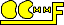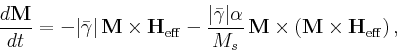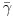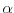Next: MIF 1.2 Up: Problem Specification File Formats Previous: MIF 2.2

Subsections

## MIF 1.1

The MIF 1.1 format is an older micromagnetic problem specification format used by the mmSolve 2D solvers. It is not compatible with the MIF 2.1 format used by the Oxs 3D solvers. However, the command line tool mifconvert may be used as a conversion aid; mifconvert is also called automatically by Oxs solvers when a MIF 1.x file is input to them.

A sample MIF 1.1 file is included below. The first line of a MIF file must be of the form # MIF x.y'', where x.y represents the format revision number. (The predecessor MIF 1.0 format was not included in any released version of OOMMF.)

After the format identifier line, any line ending in a backslash, \', is joined to the succeeding line before any other processing is performed. Lines beginning with a #' character are comments and are ignored. Blank lines are also ignored.

All other lines must consist of a Record Identifier followed by a parameter list. The Record Identifier is separated from the parameter list by one or more :' and/or =' characters. Whitespace and case is ignored in the Record Identifier field.

The parameter list must be a proper Tcl list. The parameters are parsed (broken into separate elements) following normal Tcl rules; in short, items are separated by whitespace, except as grouped by double quotes and curly braces. Opening braces and quotes must be whitespace separated from the preceding text. The grouping characters are removed during parsing. Any #' character that is found outside of any grouping mechanism is interpreted as a comment start character. The #' and all following characters on that line are interpreted as a comment.

Order of the records in a MIF 1.1 file is unimportant, except as explicitly stated below. If two or more lines contain the same Record Identifier, then the last one takes precedence, with the exception of Field Range records, of which there may be several active. All records are required unless listed as optional. Some of these record types are not supported by mmProbEd, however you may modify a MIF 1.1 file using any plain text editor and supply it to mmSolve2D using FileSource.

For convenience, the Record Identifier tags are organized into several groups; these groups correspond to the top-level buttons presented by mmProbEd. We follow this convention below.

### Material parameters

• # Material Name: This is a convenience entry for mmProbEd; inside the MIF 1.1 file it is a comment line. It relates a symbolic name (e.g., Iron) to specific values to the next 4 items. Ignored by solvers.
• Ms: Saturation magnetization in A/m.
• A: Exchange stiffness in J/m.
• K1: Crystalline anisotropy constant in J/m3 . If K1 > 0 , then the anisotropy axis (or axes) is an easy axis; if K1 < 0 then the anisotropy axis is a hard axis.
• Anisotropy Type: Crystalline anisotropy type; One of <uniaxial|cubic>.
• Anisotropy Dir1: Directional cosines of first crystalline anisotropy axis, taken with respect to the coordinate axes (3 numbers). Optional; Default is 1 0 0 (x-axis).
• Anisotropy Dir2: Directional cosines of second crystalline anisotropy axis, taken with respect to the coordinate axes (3 numbers). Optional; Default is 0 1 0 (y-axis).
For uniaxial materials it suffices to specify only Anisotropy Dir1. For cubic materials one must also specify Anisotropy Dir2; the third axis direction will be calculated as the cross product of the first two. The anisotropy directions will be automatically normalized if necessary, so for example 1 1 1 is valid input (it will be modified to .5774 .5774 .5774). For cubic materials, Dir2 will be adjusted to be perpendicular to Dir1 (by subtracting out the component parallel to Dir1).
• Anisotropy Init: Method to use to set up directions of anisotropy axes, as a function of spatial location; This is a generalization of the Anisotropy Dir1/2 records. The value for this record should be one of <Constant|UniformXY|UniformS2>. Constant uses the values specified for Anisotropy Dir1 and Dir2, with no dispersion. UniformXY ignores the values given for Anisotropy Dir1 and Dir2, and randomly varies the anisotropy directions uniformly in the xy-plane. UniformS2 is similar, but randomly varies the anisotropy directions uniformly on the unit sphere (S2 ). This record is optional; the default value is Constant.
• Edge K1: Anisotropy constant similar to crystalline anisotropy constant K1 described above, but applied only along the edge surface of the part. This is a uniaxial anisotropy, directed along the normal to the boundary surface. Units are J/m3 , with positive values making the surface normal an easy axis, and negative values making the surface an easy plane. The default value for Edge K1 is 0, which disables the term.
• Do Precess: If 1, then enable the precession term in the Landau-Lifshitz ODE. If 0, then do pure damping only. (Optional; default value is 1.)
• Gyratio: The Landau-Lifshitz gyromagnetic ratio, in m/(A.s). This is optional, with default value of 2.21e5. See the discussion of the Landau-Lifshitz ODE under the Damp Coef record identifier description.
• Damp Coef: The ODE solver in OOMMF integrates the Landau-Lifshitz equation [10,12], written aswhereis the Landau-Lifshitz gyromagnetic ratio (m/(A.s)),is the damping coefficient (dimensionless).
(See also the discussion of the Landau-Lifshitz-Gilbert equations in the Oxs documentation.) Hereis specified by the Damp Coef'' entry in the MIF 1.1 file. If not specified, a default value of 0.5 is used, which allows the solver to converge in a reasonable number of iterations. Physical materials will typically have a damping coefficient in the range 0.004 to 0.15. The 2D solver engine mmSolve requires a non-zero damping coefficient.

### Demag specification

• Demag Type: Specify algorithm and demagnetization kernel used to calculate self-magnetostatic (demagnetization) field. Must be one of
• ConstMag: Calculates the average field in each cell under the assumption that the magnetization is constant in each cell, using formulae from . (The other demag options calculate the field at the center of each cell.)
• 3dSlab: Calculate the in-plane field components using offset blocks of constant (volume) charge. Details are given in . Field components parallel to the z -axis are calculated using squares of constant (surface) charge on the upper and lower surfaces of the sample.
• 3dCharge: Calculate the in-plane field component using rectangles of constant (surface) charge on each cell. This is equivalent to assuming constant magnetization in each cell. The z -components of the field are calculated in the same manner as for the 3dSlab approach.
• FastPipe: Algorithm suitable for simulations that have infinite extent in the z -direction. This is a 2D version of the 3dSlab algorithm.
• None: No demagnetization. Fastest but least accurate method. :-}

All of these algorithms except FastPipe and None require that the Part Thickness (cf. the Part Geometry section) be set. Fast Fourier Transform (FFT) techniques are used to accelerate the calculations.

### Part geometry

• Part Width: Nominal part width (x -dimension) in meters. Should be an integral multiple of Cell Size.
• Part Height: Nominal part height (y -dimension) in meters. Should be an integral multiple of Cell Size.
• Part Thickness: Part thickness (z -dimension) in meters. Required for all demag types except FastPipe and None.
• Cell Size: In-plane (xy -plane) edge dimension of base calculation cell. This cell is a rectangular brick, with square in-plane cross-section and thickness given by Part Thickness. N.B.: Part Width and Part Height should be integral multiples of Cell Size. Part Width and Part Height will be automatically adjusted slightly (up to 0.01%) to meet this condition (affecting a small change to the problem), but if the required adjustment is too large then the problem specification is considered to be invalid, and the solver will signal an error.
• Part Shape: Optional. Part shape in the xy -plane; must be one of the following:
• Rectangle
The sample fills the area specified by Part Width and Part Height. (Default.)
• Ellipse
The sample (or the magnetically active portion thereof) is an ellipse inscribed into the rectangular area specified by Part Width and Part Height.
• Ellipsoid
Similar to the Ellipse shape, but the part thickness is varied to simulate an ellipsoid, with axis lengths of Part Width, Part Height and Part Thickness.
• Oval r
Shape is a rounded rectangle, where each corner is replaced by a quarter circle with radius r , where 0 < = r < = 1 is relative to the half-width of the rectangle.
• Pyramid overhang
Shape is a truncated pyramid, with ramp transition base width (overhang) specified in meters.
Shape and thickness are determined by a bitmap file, the name of which is specified as the second parameter. The overall size of the simulation is still determined by Part Width and Part Height (above); the bitmap is spatially scaled to fit those dimensions. Note that this scaling will not be square if the aspect ratio of the part is different from the aspect ratio of the bitmap.

The given filename must be accessible to the solver application. At present the bitmap file must be in either the PPM (portable pixmap), GIF, or BMP formats. (Formats other than the PPM P3 (text) format may be handled by spawning an any2ppm subprocess.)

White areas of the bitmap are interpreted as being non-magnetic (or having 0 thickness); all other areas are assumed to be composed of the material specified in the Material Parameters'' section. Thickness is determined by the relative darkness of the pixels in the bitmap. Black pixels are given full nominal thickness (specified by the Part Thickness'' parameter above), and gray pixels are linearly mapped to a thickness between the nominal thickness and 0. In general, bitmap pixel values are converted to a thickness relative to the nominal thickness by the formula 1-(R+G+B)/(3M), where R, G and B are the magnitudes of the red, green and blue components, respectively, and M is the maximum allowed component magnitude. For example, black has R=G=B=0, so the relative thickness is 1, and white has R=G=B=M, so the relative thickness is 0.

The code does not perform a complete 3D evaluation of thickness effects. Instead, the approximation discussed in  is implemented.

### Initial magnetization

• Init Mag: Name of routine to use to initialize the simulation magnetization directions (as a function of position), and routine parameters, if any. Optional, with default Random. The list of routines is long, and it is easy to add new ones. See the file oommf/app/mmsolve/maginit.cc for details. A few of the more useful routines are:
• Random
Random directions on the unit sphere. This is somewhat like a quenched thermal demagnetized state.
• Uniform theta phi
Uniform magnetization in the direction indicated by the two additional parameters, theta and phi, where the first is the angle from the z -axis (in degrees), and the second is the angle from the x -axis (in degrees) of the projection onto the xy -plane.
• Vortex
Fits an idealized vortex about the center of the sample.
• avfFile filename
The second parameter specifies an OVF/VIO (i.e., any'' vector field) file to use to initialize the magnetization. The grid in the input file will be scaled as necessary to fit the grid in the current simulation. The file must be accessible to the intended solver application.

### Experiment parameters

The following records specify the applied field schedule:
• Field Range: Specifies a range of applied fields that are stepped though in a linear manner. The parameter list should be 7 numbers, followed by optional control point (stopping criteria) specifications. The 7 required fields are the begin field Bx By Bz in Tesla, the end field Bx By Bz in Tesla, and an integer number of steps (intervals) to take between the begin and end fields (inclusive). Use as many Field Range records as necessary--they will be stepped through in order of appearance. If the step count is 0, then the end field is ignored and only the begin field is applied. If the step count is larger than 0, and the begin field is the same as the last field from the previous range, then the begin field is not repeated.

The optional control point specs determine the conditions that cause the applied field to be stepped, or more precisely, end the simulation of the magnetization evolution for the current applied field. The control point specs are specified as -type value pairs. There are 3 recognized control point types: -torque, -time, and -iteration. If a -torque pair is given, then the simulation at the current applied field is ended when |m x h| (i.e., |M x H|/Ms2 ) at all spins in the simulation is smaller than the specified -torque value (dimensionless). If a -time pair is given, then the simulation at the current field is ended when the elapsed simulation time for the current field step reaches the specified -time value (in seconds). Similarly, an -iteration pair steps the applied field when the iteration count for the current field step reaches the -iteration value. If multiple control point specs are given, then the applied field is advanced when any one of the specs is met. If no control point specs are given on a range line, then the Default Control Point Spec is used.

For example, consider the following Field Range line:

   Field Range: 0 0 0 .05 0 0  5  -torque 1e-5 -time 1e-9

This specifies 6 applied field values, (0,0,0), (0.01,0,0), (0.02,0,0), ..., (0.05,0,0) (in Tesla), with the advancement from one to the next occurring whenever |m x h| is smaller than 1e-5 for all spins, or when 1 nanosecond (simulation time) has elapsed at the current field. (If -torque was not specified, then the applied field would be stepped at 1, 2, 3 4 and 5 ns in simulation time.)

The Field Range record is optional, with a default value of 0 0 0 0 0 0 0.

• Default Control Point Spec: List of control point -type value pairs to use as stepping criteria for any field range with no control point specs. This is a generalization of and replacement for the Converge | mxh| Value record. Optional, with default -torque 1e-5.''

• Field Type: Applied (external) field routine and parameters, if any. This is optional, with default Uniform. At most one record of this type is allowed, but the Multi type may be used to apply a collection of fields. The nominal applied field (NAF) is stepped through the Field Ranges described above, and is made available to the applied field routines which use or ignore it as appropriate.
The following Field Type routines are available:
• Uniform
Applied field is uniform with value specified by the NAF.
• Ribbon relcharge x0 y0 x1 y1 height
Charge Ribbon,'' lying perpendicular to the xy -plane. Here relcharge is the charge strength relative to Ms, and (x0,y0), (x1,y1) are the endpoints of the ribbon (in meters). The ribbon extends height/2 above and below the calculation plane. This routine ignores the NAF.
• Tie rfx rfy rfz x0 y0 x1 y1 ribwidth
The points (x0,y0) and (x1,y1) define (in meters) the endpoints of the center spine of a rectangular ribbon of width ribwidth lying in the xy -plane. The cells with sample point inside this rectangle see an applied field of (rfx,rfy,rfz), in units relative to Ms. (If the field is large, then the magnetizations in the rectangle will be tied'' to the direction of that field.) This routine ignores the NAF.
• OneFile filename multiplier
Read B field (in Tesla) in from a file. Each value in the file is multiplied by the multiplier'' value on input. This makes it simple to reverse field direction (use -1 for the multiplier), or to convert H fields to B fields (use 1.256637e-6). The input file may be any of the vector field file types recognized by mmDisp. The input dimensions will be scaled as necessary to fit the simulation grid, with zeroth order interpolation as necessary. This routine ignores the NAF.
• FileSeq filename procname multiplier
This is a generalization of the OneFile routine that reads in fields from a sequence of files. Here filename'' is the name of a file containing Tcl code to be sourced during problem initialization, and procname'' is the name of a Tcl procedure defined in filename, which takes the nominal B field components (in Tesla) and field step count values as imports (4 values total), and returns the name of the vector field file that should be used as the applied B field for that field step. The B field units in the vector field file should be Tesla.
• Multi routinecount \
param1count name1 param1 param2 ...\
param2count name2 param1 param2 ...\
...

Allows a conglomeration of several field type routines. All entries must be on the same logical line, i.e., end physical lines with '\' continuation characters as necessary. Here routinecount is the number of routines, and param1count is the number parameters (including name1) needed by the first routine, etc.
Note that all lengths are in meters. The coordinates in the simulation lie in the first octant, running from (0,0,0) to (Part Width, Part Height, Part Thickness).

### Output specification

• Base Output Filename: Default base name used to construct output filenames.
• Magnetization Output Format: Format to use in the OVF data block for exported magnetization files. Should be one of binary 4'' (default), binary 8'', or text format-spec'', where format-spec is a C printf-style format code, such as %# .17g''. Optional.
• Total Field Output Format: Analogous to the Magnetization Output Format, but for total field output files. Optional, with default binary 4''.
• Data Table Output Format: Format to use when producing data table style scalar output, such as that sent to mmDataTable, mmGraph, and mmArchive. Should specify a C printf-style format code, such as the default %.16g''. Optional.

### Miscellaneous

• Converge | mxh| Value: Nominal value to use as a stopping criterion: When |m x h| (i.e., |M x H|/Ms2 ) at all spins in the simulation is smaller than this value, it is assumed that a relaxed (equilibrium) state has been reached for the current applied field. This is a dimensionless value.
NOTE: This Record Identifier is deprecated. Use Default Control Point Spec instead.
• Randomizer Seed: Value with which to seed random number generator. Optional. Default value is 0, which uses the system clock to generate a semi-random seed.
• Max Time Step: Limit the maximum ODE step size to no larger than this amount, in seconds. Optional.
• Min Time Step: Limit the minimum ODE step size to no less than this amount, in seconds. Optional.
• User Comment: Free-form comment string that may be used for problem identification. Optional.

# MIF 1.1
#
# Example from the OOMMF User's Guide.
#
# All units are SI.
#
################# MATERIAL PARAMETERS ######################
Ms:  800e3                # Saturation magnetization in A/m.
A:   13e-12               # Exchange stiffness in J/m.
K1:  0.5e3                # Anisotropy constant in J/m^3.
Anisotropy Type: uniaxial # One of <uniaxial|cubic>.
Anisotropy Dir1: 1 0 0    # Directional cosines wrt to
# coordinate axes

################# DEMAG SPECIFICATION ######################
Demag Type: ConstMag # One of <ConstMag|3dSlab|2dSlab
#         |3dCharge|FastPipe|None>.

#################### PART GEOMETRY #########################
Part Width:     0.25e-6    # Nominal part width in m
Part Height:    1.0e-6     # Nominal part height in m
Part Thickness: 1e-9       # Part thickness in m.
Cell Size:      7.8125e-9  # Cell size in m.
#Part Shape:    # One of <Rectangle|Ellipse|Oval|Mask>.
# Optional.

################ INITIAL MAGNETIZATION #####################
Init Mag: Uniform 90 45 # Initial magnetization routine
# and parameters

################ EXPERIMENT PARAMETERS #####################
# Field Range:  Start_field  Stop_field  Steps
Field Range: -.05 -.01 0.  .05  .01 0. 100
Field Range:  .05  .01 0. -.05 -.01 0. 100
Field Type: Multi 4 \
7 Ribbon 1 0 1.0e-6 0.25e-6 1.0e-6 1e-9 \
7 Ribbon 1 0 0      0.25e-6 0      1e-9 \
9 Tie 100 0 0 0.12e-6 0.5e-6 0.13e-6 0.5e-6 8e-9 \
1 Uniform
# The above positions ribbons of positive charge along the
# upper and lower edges with strength Ms, applies a large
# (100 Ms) field to the center cells, and also applies a
# uniform field across the sample stepped from
# (-.05,-.01,0.) to (.05,.01,0.) (Tesla), and back, in
# approximately 0.001 T steps.

Default Control Point Spec: -torque 1e-6
# Assume equilibrium has been reached, and step the applied
# field, when the reduced torque |mxh| drops below 1e-6.

################ OUTPUT SPECIFICATIONS #####################
Base Output Filename: samplerun
Magnetization Output Format: binary 8 # Save magnetization
# states in binary format with full (8-byte) precision.

#################### MISCELLANEOUS #########################
Randomizer Seed: 1   # Random number generator seed.
User Comment: Example MIF 1.1 file, with lots of comments.

Figure 9: Example MIF 1.1 file. (Description.)OOMMF Documentation Team
September 28, 2012